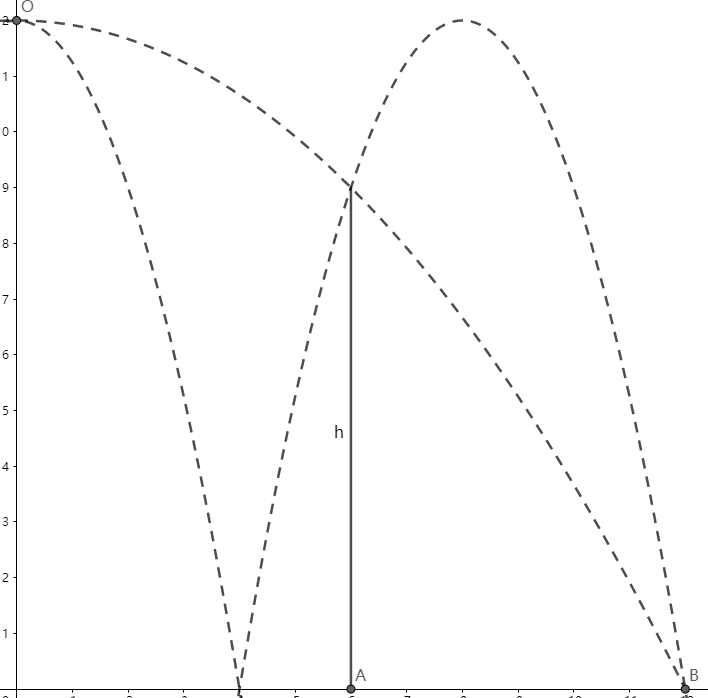# Reach for the Summit - P-S1-A3As shown above, the point $O$ is $H\text{ m}$ away from the ground. Two balls $1$ and $2$ are thrown at point $O$ horizontally, so that ball $1$ merely passes the top of the fence and falls at point $B$, ball $2$ is bounced by the ground once and then merely passes the top of the fence and falls at point $B$.

If the collision of ball $2$ and ground is elastic, find the height of the fence $h\text{ m}$.

The result is $h=\lambda H$, submit $\lfloor 1000\lambda \rfloor$.

Reach for the Summit problem set - Physics

×